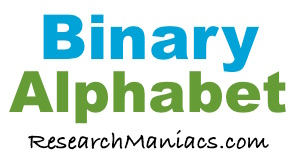Binary AlphabetHere is the 8-bit Binary Alphabet. It includes all the letters in the alphabet in both lowercase and uppercase.

 A = 01000001 B = 01000010 C = 01000011 D = 01000100 E = 01000101 F = 01000110 G = 01000111 H = 01001000 I = 01001001 J = 01001010 K = 01001011 L = 01001100 M = 01001101 N = 01001110 O = 01001111 P = 01010000 Q = 01010001 R = 01010010 S = 01010011 T = 01010100 U = 01010101 V = 01010110 W = 01010111 X = 01011000 Y = 01011001 Z = 01011010 a = 01100001 b = 01100010 c = 01100011 d = 01100100 e = 01100101 f = 01100110 g = 01100111 h = 01101000 i = 01101001 j = 01101010 k = 01101011 l = 01101100 m = 01101101 n = 01101110 o = 01101111 p = 01110000 q = 01110001 r = 01110010 s = 01110011 t = 01110100 u = 01110101 v = 01110110 w = 01110111 x = 01111000 y = 01111001 z = 01111010

If you need to convert letters or words to Binary, you could find the letters you need using the Binary Alphabet above.

However, for your convenience, we have created this Convert to Binary Tool below. You can simply enter letters or words and we will then use the Binary Alphabet above to convert your letters and words to Binary for you.

More information from Research Maniacs:

Alphabets
There are many kinds of alphabets. Go here to learn about other alphabets.

Binary Information
Do you want to learn more about Binary? Check out all our Binary information here!

Copyright  |   Privacy Policy  |   Disclaimer  |   Contact